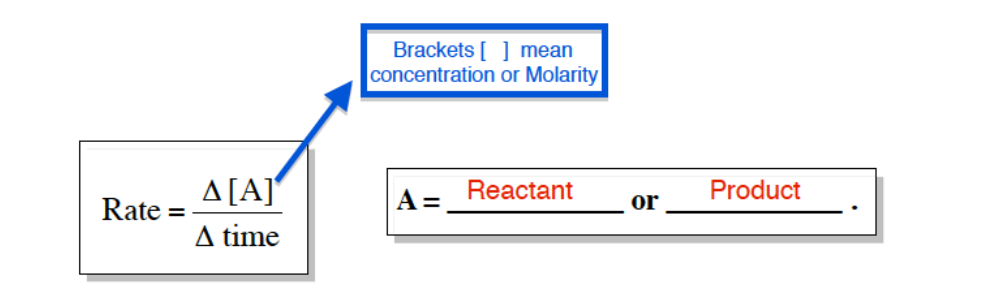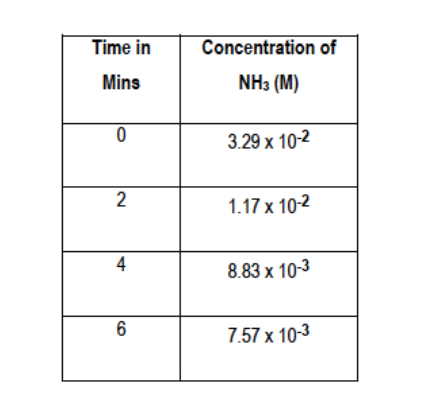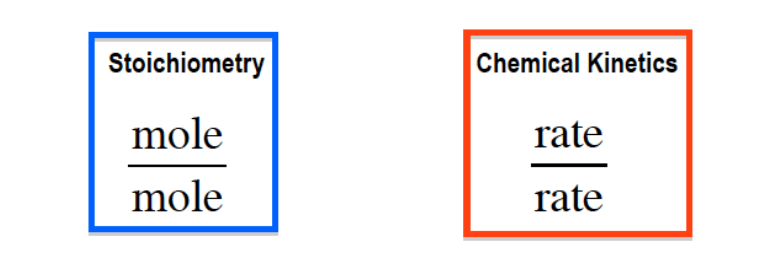Ch.13 - Chemical KineticsWorksheetSee all chapters
 Ch.1 - Intro to General Chemistry 2hrs & 53mins 0% complete Worksheet Ch.2 - Atoms & Elements 2hrs & 49mins 0% complete Worksheet Ch.3 - Chemical Reactions 3hrs & 25mins 0% complete Worksheet BONUS: Lab Techniques and Procedures 1hr & 38mins 0% complete Worksheet BONUS: Mathematical Operations and Functions 47mins 0% complete Worksheet Ch.4 - Chemical Quantities & Aqueous Reactions 3hrs & 30mins 0% complete Worksheet Ch.5 - Gases 3hrs & 47mins 0% complete Worksheet Ch.6 - Thermochemistry 2hrs & 28mins 0% complete Worksheet Ch.7 - Quantum Mechanics 2hrs & 35mins 0% complete Worksheet Ch.8 - Periodic Properties of the Elements 1hr & 57mins 0% complete Worksheet Ch.9 - Bonding & Molecular Structure 2hrs & 5mins 0% complete Worksheet Ch.10 - Molecular Shapes & Valence Bond Theory 1hr & 31mins 0% complete Worksheet Ch.11 - Liquids, Solids & Intermolecular Forces 3hrs & 40mins 0% complete Worksheet Ch.12 - Solutions 2hrs & 17mins 0% complete Worksheet Ch.13 - Chemical Kinetics 2hrs & 22mins 0% complete Worksheet Ch.14 - Chemical Equilibrium 2hrs & 26mins 0% complete Worksheet Ch.15 - Acid and Base Equilibrium 4hrs & 42mins 0% complete Worksheet Ch.16 - Aqueous Equilibrium 3hrs & 48mins 0% complete Worksheet Ch. 17 - Chemical Thermodynamics 1hr & 44mins 0% complete Worksheet Ch.18 - Electrochemistry 2hrs & 58mins 0% complete Worksheet Ch.19 - Nuclear Chemistry 1hr & 33mins 0% complete Worksheet Ch.20 - Organic Chemistry 3hrs 0% complete Worksheet Ch.22 - Chemistry of the Nonmetals 2hrs & 1min 0% complete Worksheet Ch.23 - Transition Metals and Coordination Compounds 1hr & 54mins 0% complete Worksheet

# Average Rate of Reaction

See all sections
Sections
Rate of Reaction
Average Rate of Reaction
Arrhenius Equation
Rate Law
Integrated Rate Law
Collision Theory
###### General Rate

Concept #1: Understanding Average or General Rate.

Transcript

Hey guys! In this brand new video, we're going to take a look at general rate. We've been talking about chemical kinetics this whole time. We said kinetics is just looking at the rate or speed of a chemical reaction. We're going to say general rate is the simplest way to help us explain what exactly rate is. We're going to say general rate is just looking at the change in the amount of a compound whether it’d be a product or reactant over time.
Generally, this amount is in concentration which means molarity. Here we're going to say rate equals delta A, remember delta just means change so the change in A over the change in time. What exactly does A mean? We said it already. A equals reactants or products. We're going to say this semester, the use of brackets, brackets fundamentally mean concentration. What exactly does concentration mean? Concentration means molarity.
Just in case you don't know what molarity is, remember molarity is the moles of solute over liters of solution. If you see brackets around something, it means the concentration of that compound whether it’d be a product or a reactant. Remember, solute is the smaller portion that gets dissolved inside of our solvent. The liquid itself that's doing the dissolving is the solvent. The smaller portion is the solute. Solute plus solvent equals solution.

General or Average Rate is the change in the concentration of a compound over a period of time.Example #1: The following equation shows the production of NO and H2O by oxidation of ammonia.

4 NH3 (g) +  5 O2 (g)   →  4 NO (g)  + 6 H2O (l)

a. What is the average rate of each compound in the balanced equation?Example #2: b) What is the rate of NH3 in the reaction between 2 and 6 minutes at 40oC?

Example #3: c) Determine the instantaneous rate of the following reaction.

###### Stoichiometric Rate

In a stoichiometry based question we are accustomed to doing a mole-to-mole comparison. Now in Chemical Kinetics, we will do instead a rate-to-rate comparison.Example #4: The decomposition of dinitrogen pentoxide is described by the chemical equation:

2 N2O5 (g)   --->  4 NO2 (g)  +  O2 (g)

If the rate of disappearance or decomposition of O2 is equal to 2.20 M/min at a particular moment, what is the rate of appearance or formation of N2O5 at that moment?

Practice: The formation of alumina, Al2O3, can be illustrated by the reaction below: 4 Al (s) + 3 O2 (g) ----> 2 Al2O3 (s). At 750 K it takes 267 seconds for the initial concentration of Al2O3 to increase from 6.18 E-5 M to 5.11 E-4 M. What is the rate of Al?